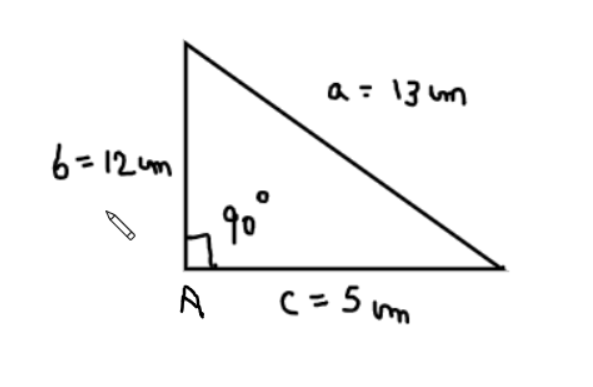Courses
Courses for Kids
Free study material
Free LIVE classes
MoreLIVE
Join Vedantu’s FREE Mastercalss

# If ${\text{a = 13,b = 12,c = 5}}$in $\vartriangle {\text{ABC}}$where a, b, c are the sides of a triangle, then the value of ${\text{sin}}\dfrac{A}{2} =$$\begin{gathered} \left( a \right){\text{ }}\dfrac{1}{{\sqrt 5 }} \\ \left( b \right){\text{ }}\dfrac{2}{3} \\ \left( c \right){\text{ }}\sqrt {\dfrac{{32}}{{35}}} \\ \left( d \right){\text{ }}\dfrac{1}{{\sqrt 2 }} \\ \end{gathered}$Verified
366k+ views
Hint- Try to figure out whether the triangular sides formulated above forms a right angled triangle or not.In the above figure we can see that $\vartriangle {\text{ABC}}$has the sides ${\text{a = 13,b = 12 and c = 5}}$
Now if we try to apply Pythagoras theorem which states that if ${\text{hypotenuse}}{{\text{s}}^2} = {\text{perpendicular}}{{\text{r}}^2} + {\text{bas}}{{\text{e}}^2}$then the triangle will be a right angle triangle.
Thus clearly ${{\text{a}}^2} = {{\text{b}}^2} + {{\text{c}}^2}$that is ${\text{1}}{{\text{3}}^2} = {12^2} + {5^2}$or ${\text{169 = 144 + 25}}$
Hence we can say that the above triangle is a right angle triangle and from the above figure it is clear that it is right angled at A that is$\angle {\text{A = 90}}$.
Let’s discuss why it is right angled at A only and not B or C?
Because $\angle {\text{A}}$is the opposite angle to the greatest side of the triangle and Pythagoras theorem is also applicable.
Hence ${\text{sin}}\dfrac{A}{2} = \sin \dfrac{{90}}{2} = \sin 45 = \dfrac{1}{{\sqrt 2 }}$
Hence option (d) is the correct option.
Note-If a triangle is found obeying the Pythagoras theorem then the angle which is always opposite to the greatest side is 90 degree or in other words if a triangle is obeying Pythagoras theorem than it is right angled at the angle which is exactly opposite to the greatest side in that triangle.
Last updated date: 29th Sep 2023
Total views: 366k
Views today: 8.66k